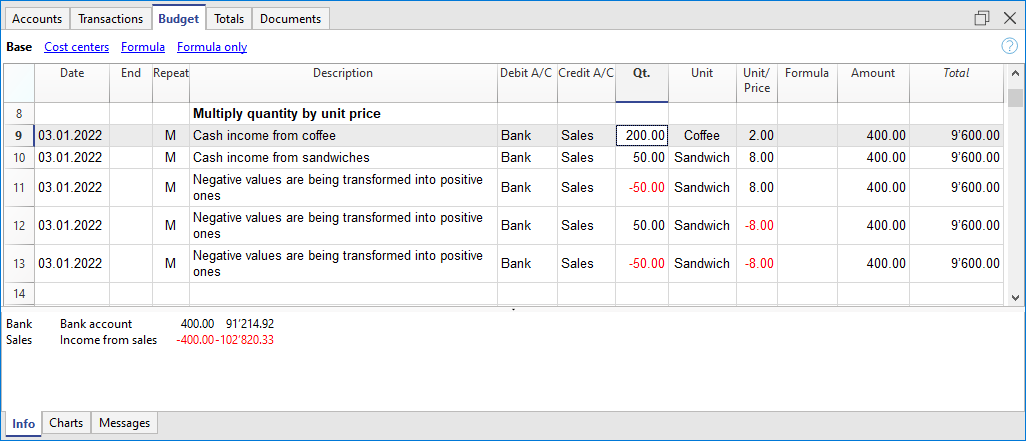# Forecasting with quantity and price

Documentation •

The Quantity, Unit and Unit Price columns of the Budget table allow you to prepare forecasts faster.

The value of the Amount column is calculated by the program by multiplying the Quantity by the Unit Price (under the condition that Formula column is empty).

The Quantity, Unit Price columns are set as visible in the Formula View.## Advantages of using the Quantity and Prices columns

The quantity and price columns are useful for making predictions based on quantities. For example:

• In the Unit column you can indicate what the price refers to.
• The Quantity column indicates the number of seats served daily.
• The Price column indicates the estimated revenue for each cover.
• The Amount column will be calculated automatically based on the values indicated.

• All elements of the planning can be precisely detailed in the Budget.
• We remember the quantities and prices used to make the estimate.
• Changing the schedule is very simple, you can change the element you want only.
• How the profit varies, can be seen with a change in the quantities sold or in the price.
Break-even analysis.

help_id
Forecasting quantity price